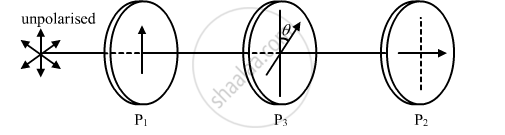# Two polaroids P1 and P2 are placed with their pass axes perpendicular to each other. Unpolarised light of intensity I0 is incident on P1. - Physics

Two polaroids P1 and P2 are placed with their pass axes perpendicular to each other. Unpolarised light of intensity I0 is incident on P1. A third polaroid P3 is kept in between P1 and P2 such that its pass axis makes an angle of 60° with that of P1. Determine the intensity of light transmitted through P1, P2 and P3.

A narrow beam of unpolarised light of intensity I0 is incident on a polaroid P1. The light transmitted by it is then incident on a second polariod P2 with its pass axis making angle of 60° relative to the pass axis of P1. Find the intensity of the light transmitted by P2.

#### Solution

As given in the question the polaroid P1 and P2 are placed with their pass axes perpendicular to each other and polaroid P3 placed at 60°with repect to P1.Therefore,
Intensity of light after falling on first polaroid  P1I'=I_0/2

Intensity of light after falling on third polaroid P3

I'' = I'cos^2(theta)=I_0/2cos^2(60^@)=I_0/8

Therefore the intensity I_0/8 will pass through the P3 and angle between P3 and P2 is 30°. because of the condition given in the question.

Intensity of light after falling on second polaroid P=

I'''= I'' cos^2(theta)=I_0/8cos^2(30^@) = (3I_0)/32

Concept: Polarisation
Is there an error in this question or solution?
2013-2014 (March) All India Set 3

Share